MORE IN Network Analysis
Total marks: --
Total time: --
INSTRUCTIONS
(1) Assume appropriate data and state your reasons
(2) Marks are given to the right of every question
(3) Draw neat diagrams wherever necessary

1 (a) Find the equivalent resistance at AB using Y-Δ transformation technique for the circuit shown in fig. Q1(a). (All the resistors connected are 30 Ω each).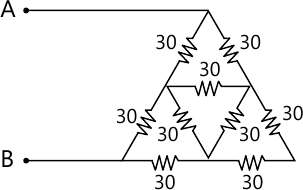5 M
1 (b) Find 'is' and 'vx' for the circuit shown in Fig Q1(b) by Mesh analysis.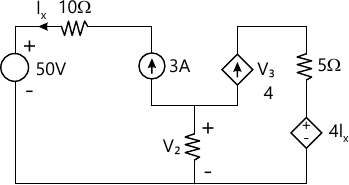5 M
1 (c) For the network given in Fig Q1(c). Find 'In' using nodal analysis.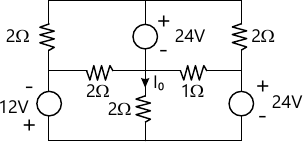10 M

2 (a) Write the tie-set schedule for the network shown in Fig. Q2(a), and using the tie set schedule determine all branch currents. ( Take resistors as tree branch elements).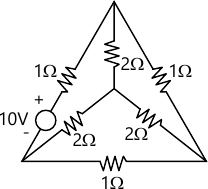10 M
2 (b) For the network shown in Fig Q2(b), draw the dual circuit. Also write the nodal equations for the dual circuit.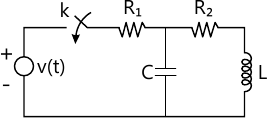10 M

3 (a) Find the voltage 'V' across 3Ω resistor using superposition theorems for the circuit given in the fig. Q3 (a)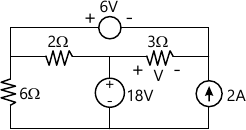10 M
3 (b) State Millman's theorem. Using Millman's theorem find current through the load resistor R1 for the circuit shown in Fig. Q3(b).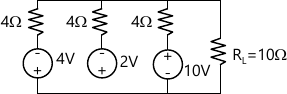10 M

4 (a) Find the Thevenin's equivalent of the network shown in Fig. Q4(a).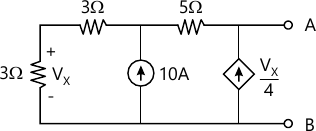10 M
4 (b) State maximum power transfer theorem. For the circuit shown in Fig. Q4(b), what should be the value of 'R' such that maximum power transfer can take place from the rest of the network to 'R'. Obtain the amount of this power.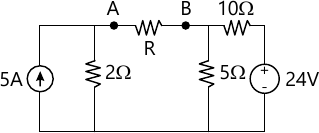10 M

5 (a) A 220 V, 100 Hz AC source supplies a series RLC circuit with a capacitor and a coil. If the coil has 50m Ω resistance and 5 mH inductance, find at a resonance frequency of 100 Hz what is the value of capacitor. Also calculate the Q factor half power frequencies of the circuit.
10 M
5 (b) Find the value of R1 such that the circuit given in Fig Q5(b) is resonant.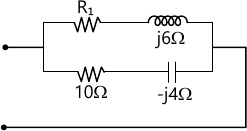6 M
5 (c) Determine R1 and R0 that causes the circuit to be resonant at all frequencies for the circuit shown in Fig. Q5(c).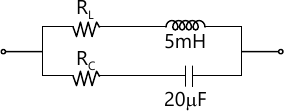4 M

6 (a) In the network shown in fig Q6(a) the switch is closed at t=0. Determine $i, \dfrac {d_i}{d_t} \ and \ \dfrac{d^2 i}{d _t^2}\ at \ t=0 '$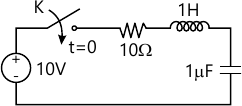10 M
6 (b) For the circuit shown in Fig Q6(b), the switch 'K' is changed from position -1 to position 2 at t=0 steady=state condition having been reached at position =1. Find the value of $i, \dfrac {d_i}{d_t}\cdot \dfrac {d^2i}{dt^2} \ at \ t=0'$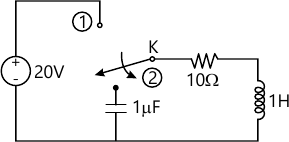10 M

7 (a) In the Fig Q7(a), the battery voltage '10'V is applied for a steady period with switch 'K' open. Open the complete expression for the current after closing the switch K. Use Laplace transforms.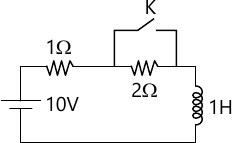10 M
7 (b) Referring to the Fig. Q7(b). Solve for i(t). using Laplace transformation.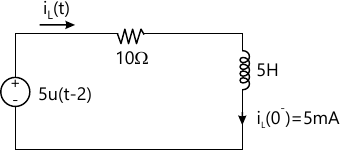10 M

8 (a) Find the 'z' parameters of the circuit shown in Fig. 8(a).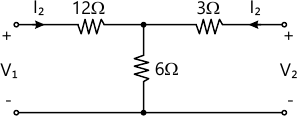10 M
8 (b) Following are the hybrid parameters for a network $\begin{bmatrix} h_{11} &h_{12} \\h_{21} &h_{22} \end{bmatrix} = \begin{bmatrix} 5 &2 \\3 &6 \end{bmatrix}$ Determine the Y parameter for the network.
10 M

More question papers from Network Analysis